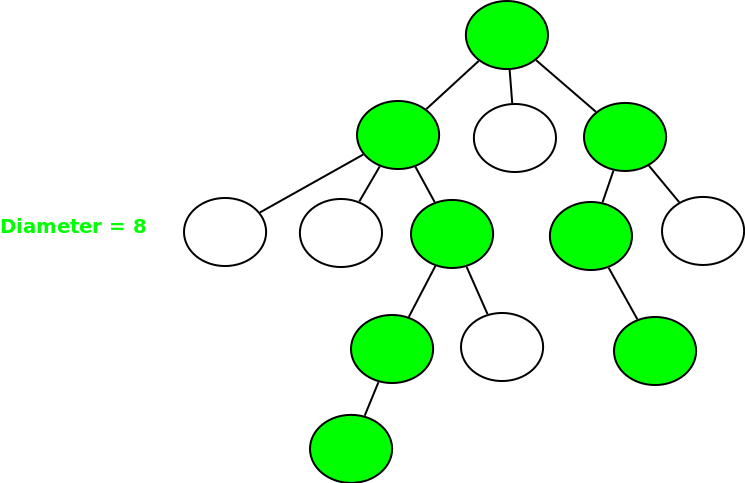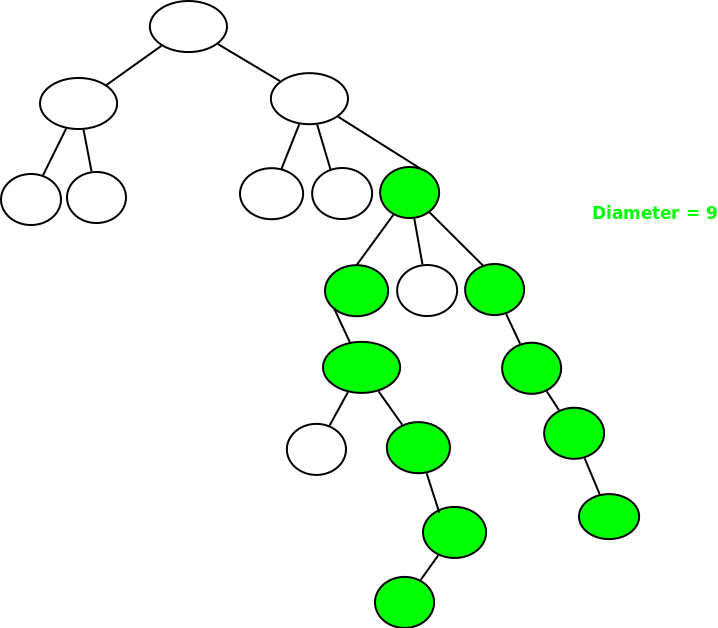# Diameter of an N-ary tree

The diameter of an N-ary tree is the longest path present between any two nodes of the tree. These two nodes must be two leaf nodes. The following examples have the longest path[diameter] shaded.

Examples:Example 2:## Recommended: Please try your approach on {IDE} first, before moving on to the solution.

Prerequisite : Diameter of a binary tree.

The path can either start from one of the node and goes up to one of the LCAs of these nodes and again come down to the deepest node of some other subtree or can exist as a diameter of one of the child of the current node.
The solution will exist in any one of these:
I] Diameter of one of the children of the current node
II] Sum of Height of highest two subtree + 1

 `// C++ program to find the height of an N-ary ` `// tree ` `#include ` `using` `namespace` `std; ` ` `  `// Structure of a node of an n-ary tree ` `struct` `Node ` `{ ` `    ``char` `key; ` `    ``vector child; ` `}; ` ` `  `// Utility function to create a new tree node ` `Node *newNode(``int` `key) ` `{ ` `    ``Node *temp = ``new` `Node; ` `    ``temp->key = key; ` `    ``return` `temp; ` `} ` ` `  `// Utility function that will return the depth ` `// of the tree ` `int` `depthOfTree(``struct` `Node *ptr) ` `{ ` `    ``// Base case ` `    ``if` `(!ptr) ` `        ``return` `0; ` ` `  `    ``int` `maxdepth = 0; ` ` `  `    ``// Check for all children and find ` `    ``// the maximum depth ` `    ``for` `(vector::iterator it = ptr->child.begin(); ` `                           ``it != ptr->child.end(); it++) ` ` `  `        ``maxdepth = max(maxdepth , depthOfTree(*it)); ` ` `  `    ``return` `maxdepth + 1; ` `} ` ` `  `// Function to calculate the diameter ` `// of the tree ` `int` `diameter(``struct` `Node *ptr) ` `{ ` `    ``// Base case ` `    ``if` `(!ptr) ` `        ``return` `0; ` ` `  `    ``// Find top two highest children ` `    ``int` `max1 = 0, max2 = 0; ` `    ``for` `(vector::iterator it = ptr->child.begin(); ` `                          ``it != ptr->child.end(); it++) ` `    ``{ ` `        ``int` `h = depthOfTree(*it); ` `        ``if` `(h > max1) ` `           ``max2 = max1, max1 = h; ` `        ``else` `if` `(h > max2) ` `           ``max2 = h; ` `    ``} ` ` `  `    ``// Iterate over each child for diameter ` `    ``int` `maxChildDia = 0; ` `    ``for` `(vector::iterator it = ptr->child.begin(); ` `                           ``it != ptr->child.end(); it++) ` `        ``maxChildDia = max(maxChildDia, diameter(*it)); ` ` `  `    ``return` `max(maxChildDia, max1 + max2 + 1); ` `} ` ` `  `// Driver program ` `int` `main() ` `{ ` `    ``/*   Let us create below tree ` `    ``*           A ` `    ``*         / /  \  \ ` `    ``*       B  F   D  E ` `    ``*      / \     |  /|\ ` `    ``*     K  J    G  C H I ` `    ``*      /\            \ ` `    ``*    N   M            L ` `    ``*/` ` `  `    ``Node *root = newNode(``'A'``); ` `    ``(root->child).push_back(newNode(``'B'``)); ` `    ``(root->child).push_back(newNode(``'F'``)); ` `    ``(root->child).push_back(newNode(``'D'``)); ` `    ``(root->child).push_back(newNode(``'E'``)); ` `    ``(root->child->child).push_back(newNode(``'K'``)); ` `    ``(root->child->child).push_back(newNode(``'J'``)); ` `    ``(root->child->child).push_back(newNode(``'G'``)); ` `    ``(root->child->child).push_back(newNode(``'C'``)); ` `    ``(root->child->child).push_back(newNode(``'H'``)); ` `    ``(root->child->child).push_back(newNode(``'I'``)); ` `    ``(root->child->child->child).push_back(newNode(``'N'``)); ` `    ``(root->child->child->child).push_back(newNode(``'M'``)); ` `    ``(root->child->child->child).push_back(newNode(``'L'``)); ` ` `  `    ``cout << diameter(root) << endl; ` ` `  `    ``return` `0; ` `} `

Output:

```7
```

Optimizations to above solution :
We can make a hash table to store heights of all nodes. If we precompute these heights, we don’t need to call depthOfTree() for every node.

A different optimized solution :
Longest path in an undirected tree

This article is contributed by Shubham Gupta. If you like GeeksforGeeks and would like to contribute, you can also write an article using contribute.geeksforgeeks.org or mail your article to contribute@geeksforgeeks.org. See your article appearing on the GeeksforGeeks main page and help other Geeks.

My Personal Notes arrow_drop_up

Article Tags :
Practice Tags :

4

Please write to us at contribute@geeksforgeeks.org to report any issue with the above content.